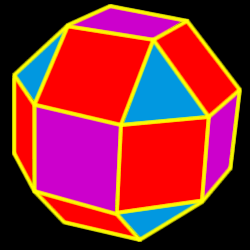# Rhombicuboctahedron

Properties of the rhombicuboctahedron: Number of faces, edges and dihedral angle measure

The rhombicuboctahedron is created by truncating (cutting off) the cuboctahedron one half of the way into each side.26 total faces:
8 equilateral triangles and 18 squares

24 vertices:
1 triangle and 3 squares

48 edges

Dihedral angles:
144 degrees, 44 minutes for the sqr-tri angle and 135 degrees for the sqr-sqr angle